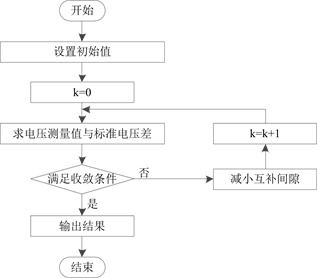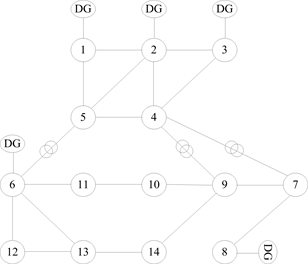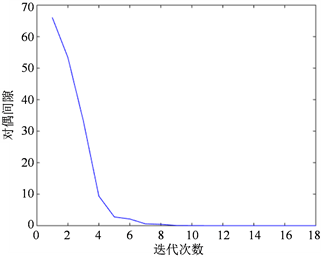﻿ 基于原对偶内点法的新能源配电网无功优化模型

# 基于原对偶内点法的新能源配电网无功优化模型Reactive Power Optimization Model for Distribution Networks with Renewable En-ergy Based on Primal-Dual Interior Point Algorithm

Abstract: The uncertainty of the distributed power supply’s output has a major impact on the voltage fluc-tuation of power system, the power flow distribution and the power quality. Considering the problems such as the voltage fluctuation of distribution network and the over limit, for the pur-pose of regulating the voltage, improving the power quality and decreasing the system loss, a new method named second-order cone programming method proposed in this article can solve the reactive power optimization problem based on complex nonlinear programming problem in distribution network which includes the distributed power supply. Firstly, the new method which takes the voltage deviation of each node in power system and the distributed power supply’s output limit as constraints and takes the access location and the access capacity as decision variable establishes power system SOCP model aimed at minimizing the loss of power system. Then the article solves the problem using Modern primal dual interior point algorithm. The validity and feasibility of the proposed model and method are verified by the calculation results of IEEE14 system. It also shows that the method has high computational efficiency and feasibility.

1. 引言

2. 原对偶内点算法

2.1. 原始对偶内点法基本原理

$\begin{array}{l}\mathrm{min}\text{\hspace{0.17em}}\text{\hspace{0.17em}}f\left(x\right)\\ \text{s}\text{.t}\text{.}\text{\hspace{0.17em}}\text{\hspace{0.17em}}\text{\hspace{0.17em}}h\left(x\right)=0\\ {g}_{\mathrm{min}} (1)

$\left\{\begin{array}{l}g-l-{g}_{\mathrm{min}}=0\hfill \\ {g}_{\mathrm{max}}-u-g=0\hfill \\ l>0,u<0\hfill \end{array}$ (2)

$\begin{array}{c}F=f\left(x\right)+{y}^{\text{T}}h\left(x\right)+{z}^{\text{T}}\left[g\left(x\right)-l-{g}_{\mathrm{min}}\right]\\ \text{\hspace{0.17em}}\text{\hspace{0.17em}}+{w}^{\text{T}}\left[g\left(x\right)+u-{g}_{\mathrm{max}}\right]-u\left(\underset{i=1}{\overset{r}{\sum }}\mathrm{ln}{l}_{i}+\underset{i=1}{\overset{r}{\sum }}\mathrm{ln}{u}_{i}\right)\end{array}$ (3)

2.2. 迭代步长的确定

$\begin{array}{l}{\alpha }_{p}=0.9995\mathrm{min}\left[\mathrm{min}\left(\frac{-{l}_{i}}{\Delta {l}_{i}},\Delta {l}_{i}<0;\frac{-{u}_{i}}{\Delta {u}_{i}},\Delta {u}_{i}<0\right),1\right]\\ {\alpha }_{q}=0.9995\mathrm{min}\left[\mathrm{min}\left(\frac{-{z}_{i}}{\Delta {z}_{i}},\Delta {l}_{i}<0;\frac{-{w}_{i}}{\Delta {w}_{i}},\Delta {w}_{i}<0\right),1\right]\end{array}$ (4)

2.3. 障碍常数的确定

$Gap=\underset{i=1}{\overset{r}{\sum }}\left({u}_{r}{w}_{r}-{l}_{r}{z}_{r}\right)$ (5)

3. 配电网无功优化的锥规划模型

3.1. 锥规划

$\mathrm{min}\left\{{c}^{\text{T}}x:Ax=b,x\ge 0\right\}$ (6)

$\mathrm{max}\left\{{b}^{\text{T}}y:{A}^{\text{T}}y+s=c,s\ge 0\right\}$ (7)

$\left\{\begin{array}{l}\begin{array}{ll}Ax=b\hfill & x\ge 0\hfill \\ {A}^{\text{T}}y+s=c\hfill & s\ge 0\hfill \end{array}\\ xs=0\end{array}$ (8)

$\mathrm{min}\left\{{\left(c-v{r}_{c}^{0}\right)}^{\text{T}}x:Ax=b-v{r}_{b}^{0},x\ge 0\right\}$ (9)

$\mathrm{max}\left\{{\left(b-v{r}_{b}^{0}\right)}^{\text{T}}x:{A}^{T}{y}^{0}+s=c-v{r}_{c}^{0},s\ge 0\right\}$ (10)

$\left\{\begin{array}{l}\begin{array}{ll}b-Ax=v{r}_{b}^{0}\hfill & x\ge 0\hfill \\ c-{A}^{\text{T}}y-s=v{r}_{c}^{0}\hfill & s\ge 0\hfill \end{array}\\ xs=ue\end{array}$ (11)

3.2. 无功优化数学模型

$\begin{array}{l}\mathrm{min}f\left(x\right)=\underset{i=1}{\overset{n}{\sum }}\underset{j\in i}{\sum }{G}_{ij}\left({V}_{i}^{2}+{V}_{j}^{2}-2{V}_{i}{V}_{j}\mathrm{cos}{\theta }_{ij}\right)\\ {P}_{Gi}-{P}_{Li}-{V}_{i}\underset{j=1}{\overset{n}{\sum }}{V}_{j}\left({G}_{ij}\mathrm{cos}{\theta }_{ij}+{B}_{ij}\mathrm{sin}{\theta }_{ij}\right)\\ {Q}_{Gi}-{Q}_{Ci}-{Q}_{Li}-{V}_{i}\underset{j=1}{\overset{n}{\sum }}{V}_{j}\left({G}_{ij}\mathrm{sin}{\theta }_{ij}-{B}_{ij}\mathrm{cos}{\theta }_{ij}\right)=0\\ {V}_{\mathrm{min}}<{V}_{i}<{V}_{\mathrm{max}}\\ {Q}_{Gi\mathrm{min}}<{Q}_{Gi}<{Q}_{Gi\mathrm{max}}\end{array}$ (12)

3.3. 等式约束的雅可比矩阵

$\begin{array}{c}{J}_{g}\left(x\right)=\left(\begin{array}{llll}\frac{\partial {g}_{P}}{\partial {P}_{G}}\hfill & \frac{\partial {g}_{P}}{\partial {Q}_{R}}\hfill & \frac{\partial {g}_{P}}{\partial V}\hfill & \frac{\partial {g}_{P}}{\partial \theta }\hfill \\ \frac{\partial {g}_{Q}}{\partial {P}_{G}}\hfill & \frac{\partial {g}_{Q}}{\partial {Q}_{R}}\hfill & \frac{\partial {g}_{Q}}{\partial V}\hfill & \frac{\partial {g}_{Q}}{\partial \theta }\hfill \end{array}\right)\\ =\left(\begin{array}{llll}{E}_{{g}_{Q}}\hfill & 0\hfill & N\hfill & H\hfill \\ 0\hfill & {E}_{{g}_{Q}}\hfill & L\hfill & K\hfill \end{array}\right)\end{array}$ (13)

${E}_{{g}_{P}}\left(x\right)={\left(\begin{array}{cccc}\frac{\partial {g}_{P1}}{\partial {P}_{G1}}& \frac{\partial {g}_{P1}}{\partial {P}_{G2}}& \cdots & \frac{\partial {g}_{P1}}{\partial {P}_{Gg}}\\ \frac{\partial {g}_{P2}}{\partial {P}_{G1}}& \frac{\partial {g}_{P2}}{\partial {P}_{G2}}& \cdots & \frac{\partial {g}_{P2}}{\partial {P}_{Gg}}\\ ⋮& ⋮& \ddots & ⋮\\ \frac{\partial {g}_{Pn}}{\partial {P}_{G1}}& \frac{\partial {g}_{Pn}}{\partial {P}_{G2}}& \cdots & \frac{\partial {g}_{Pn}}{\partial {P}_{Gg}}\end{array}\right)}_{n×g}$ (14)

${E}_{{g}_{Q}}\left(x\right)={\left(\begin{array}{cccc}\frac{\partial {g}_{Q1}}{\partial {Q}_{R1}}& \frac{\partial {g}_{Q1}}{\partial {Q}_{R2}}& \cdots & \frac{\partial {g}_{Q1}}{\partial {Q}_{Rr}}\\ \frac{\partial {g}_{Q2}}{\partial {Q}_{R1}}& \frac{\partial {g}_{Q2}}{\partial {Q}_{R2}}& \cdots & \frac{\partial {g}_{Q2}}{\partial {Q}_{Rr}}\\ ⋮& ⋮& \ddots & ⋮\\ \frac{\partial {g}_{Qn}}{\partial {Q}_{R1}}& \frac{\partial {g}_{Qn}}{\partial {Q}_{R2}}& \cdots & \frac{\partial {g}_{Qn}}{\partial {Q}_{Rr}}\end{array}\right)}_{n×r}$ (15)

$N={\left(\begin{array}{cccc}\frac{\partial {g}_{P1}}{\partial {V}_{1}}& \frac{\partial {g}_{P1}}{\partial {V}_{2}}& \cdots & \frac{\partial {g}_{P1}}{\partial {V}_{n}}\\ \frac{\partial {g}_{P2}}{\partial {V}_{1}}& \frac{\partial {g}_{P2}}{\partial {V}_{2}}& \cdots & \frac{\partial {g}_{P2}}{\partial {V}_{n}}\\ ⋮& ⋮& \ddots & ⋮\\ \frac{\partial {g}_{Pn}}{\partial {V}_{1}}& \frac{\partial {g}_{Pn}}{\partial {V}_{2}}& \cdots & \frac{\partial {g}_{Pn}}{\partial {V}_{n}}\end{array}\right)}_{n×n}$ (16)

3.4. 不等式约束的海森矩阵

${\nabla }_{x}^{2}{h}_{i}\left(x\right)=\left(\begin{array}{cccc}\frac{{\partial }^{2}{h}_{{P}_{i}}}{{\partial }^{2}{P}_{G}}& \frac{{\partial }^{2}{h}_{{P}_{i}}}{\partial {P}_{G}\partial {Q}_{R}}& \frac{{\partial }^{2}{h}_{{P}_{i}}}{\partial {P}_{G}\partial V}& \frac{{\partial }^{2}{h}_{{P}_{i}}}{\partial {P}_{G}\partial \theta }\\ \frac{{\partial }^{2}{h}_{{P}_{i}}}{\partial {Q}_{R}\partial {P}_{G}}& \frac{{\partial }^{2}{h}_{{P}_{i}}}{{\partial }^{2}{Q}_{R}}& \frac{{\partial }^{2}{h}_{{P}_{i}}}{\partial {Q}_{R}\partial V}& \frac{{\partial }^{2}{h}_{{P}_{i}}}{\partial {Q}_{R}\partial \theta }\\ \frac{{\partial }^{2}{h}_{{P}_{i}}}{\partial V\partial {P}_{G}}& \frac{{\partial }^{2}{h}_{{P}_{i}}}{\partial V\partial {Q}_{R}}& \frac{{\partial }^{2}{h}_{{P}_{i}}}{{\partial }^{2}V}& \frac{{\partial }^{2}{h}_{{P}_{i}}}{\partial V\partial \theta }\\ \frac{{\partial }^{2}{h}_{{P}_{i}}}{\partial \theta \partial {P}_{G}}& \frac{{\partial }^{2}{h}_{{P}_{i}}}{\partial \theta \partial {Q}_{R}}& \frac{{\partial }^{2}{h}_{{P}_{i}}}{\partial \theta \partial V}& \frac{{\partial }^{2}{h}_{{P}_{i}}}{{\partial }^{2}\theta }\end{array}\right)$ (17)

3.5. 原对偶内点法无功优化算法步骤

1) 设置初值(可行的初始点，惩罚因子初始值，缩减系数，收敛精度，设置初始迭代次数k = 0)；

2) 构造惩罚函数，求解目标函数极值；

3) 判断所得函数极值是否满足收敛条件，若满足则迭代终止，若不满足则缩小惩罚因子，当前极值为下次迭代初始值，直至满足收敛条件。

4. 算例分析Figure 1. Solving stepsFigure 2. IEEE14 distribution systemFigure 3. The compensation Gap varies with the number of iterationsTable 1. Optimization results of system state variables

5. 总结

DG出力的不确定性造成电网系统电压不稳定以及损耗值增大等问题，合理的调节配电网无功可以有效减少系统有功损耗、增强系统电能稳定。通过接入IEEE14节点系统比，得出原对偶内点法是可行的且具有较高的计算速度和精确度。

 王伟. 含分布式电源的配电网潮流计算及网损分析的研究[D]: [硕士学位论文]. 兰州: 兰州理工大学, 2014.

 Sheng, W., Liu, K.Y., Liu, Y., et al. (2016) Reactive Power Coordinated Optimisation Method with Renewable Distributed Generation Based on Improved Harmony Search. IET Generation Transmission & Distribution, 10, 3152-3162.
https://doi.org/10.1049/iet-gtd.2015.1051

 Billinton, R., Fotuhi-Firuzabad, M. and Chen, H. (1999) Probabilistic Performance Assessment of Wind Energy Conversion Systems. Discussion, 14, 223.

 Li, Q., Liu, M. and Liu, H. (2015) Piecewise Normalized Normal Constraint Method Applied to Minimization of Voltage Deviation and Active Power Loss in an AC-DC Hybrid Power System. IEEE Transactions on Power Systems, 30, 1243-1251.
https://doi.org/10.1109/TPWRS.2014.2343625

 李文琦. 含分布式发电的配电网网损分析[D]: [硕士学位论文]. 北京: 华北电力大学, 2012.

 Bouhouras, A.S., Sgouras, K.I., Gkaidatzis, P.A., et al. (2016) Optimal Active and Reactive Nodal Power Requirements towards Loss Minimization under Reverse Power Flow Constraint Defining DG Type. International Journal of Electrical Power & Energy Systems, 78, 445-454.
https://doi.org/10.1016/j.ijepes.2015.12.014

 赵晋泉, 居俐洁, 戴则梅, 等. 基于分支定界—原对偶内点法的日前无功优化[J]. 电力系统自动化, 2015(15): 55-60.

 Lei, M., Yang, W., Peng, X., et al. (2015) Reactive Power Optimization of the Distribution Network With Distributed Generation Based on Improved Simple PSO Algorithm. Journal of North China Electric Power University, 42, 39-44.

 常鲜戎, 张亮平, 郑焕坤. 非线性原-对偶内点法无功优化中的修正方程降维方法[J]. 电网技术, 2011, 35(5): 46-51.

 蒋平, 梁乐. 基于内点法和遗传算法相结合的交直流系统无功优化[J]. 高电压技术, 2015, 41(3): 724-729.

 Zhou, H.Q., Guo, J.Z., Zhou, R.L., Gu, K.H. and Qian, L.M. (2016) Interior Point Method Based Reactive Power Optimization of Active Distribution Network. China International Conference on Electricity Distribution, Xi’an, 10-13 August 2016, 1123-1128.

 庄园, 王磊. 分布式电源在配电网络中优化选址与定容的研究[J]. 电力系统保护与控制, 2012(20): 73-78.

 Teshome, D.F. and Lian, K.L. (2016) A Smart Distribution System Reconfiguration Algorithm with Optimal Active Power Scheduling Considering Various Types of Distributed Generators. IEEJ Transactions on Electrical & Electronic Engineering, 11, 564-576.
https://doi.org/10.1002/tee.22274

 张立溥. 锥规划的全牛顿步不可行内点算法[D]: [硕士学位论文]. 上海: 上海大学, 2011.

Top